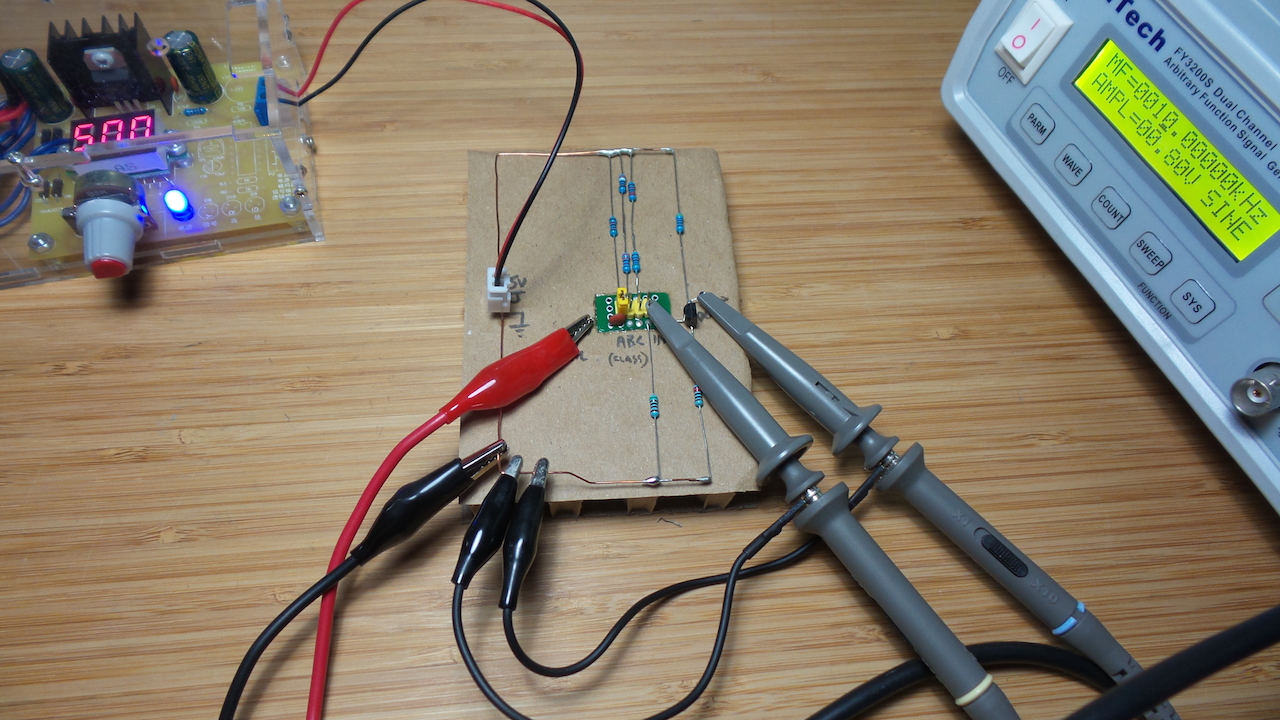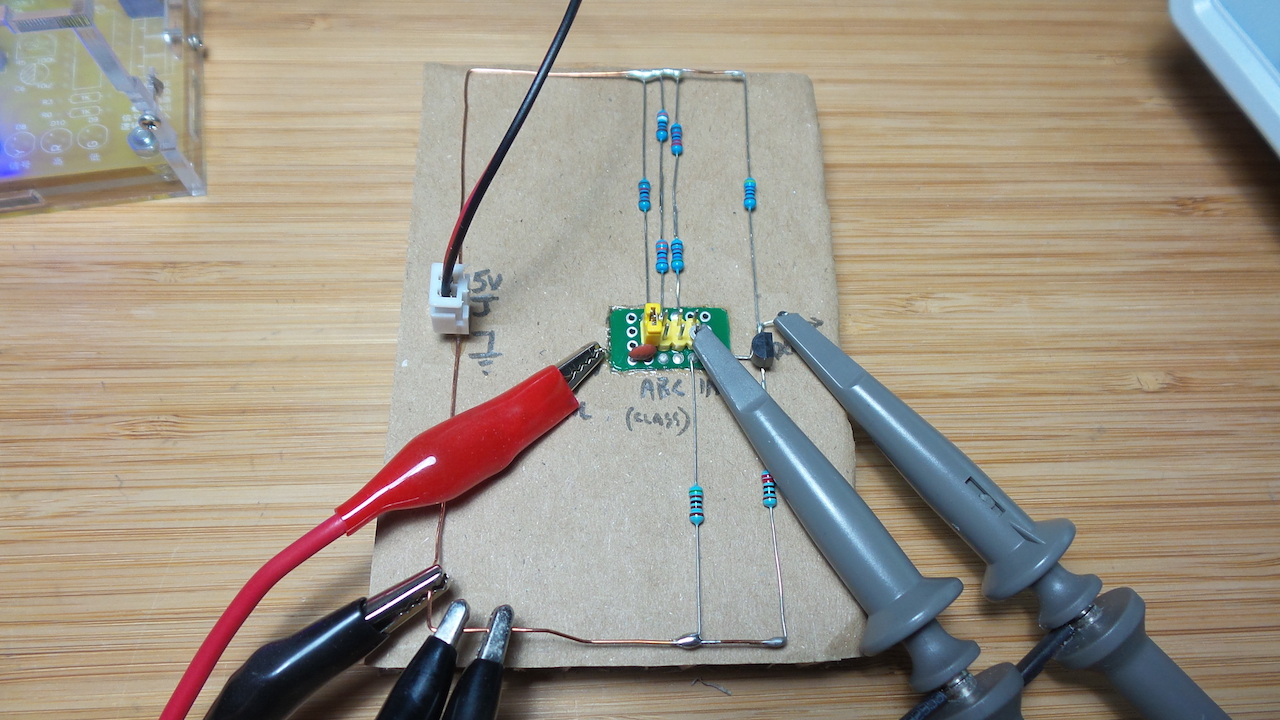# #371 BJT/CommonEmitterAmplifier

All about BJT common-emitter amplifier biasing and class of operation.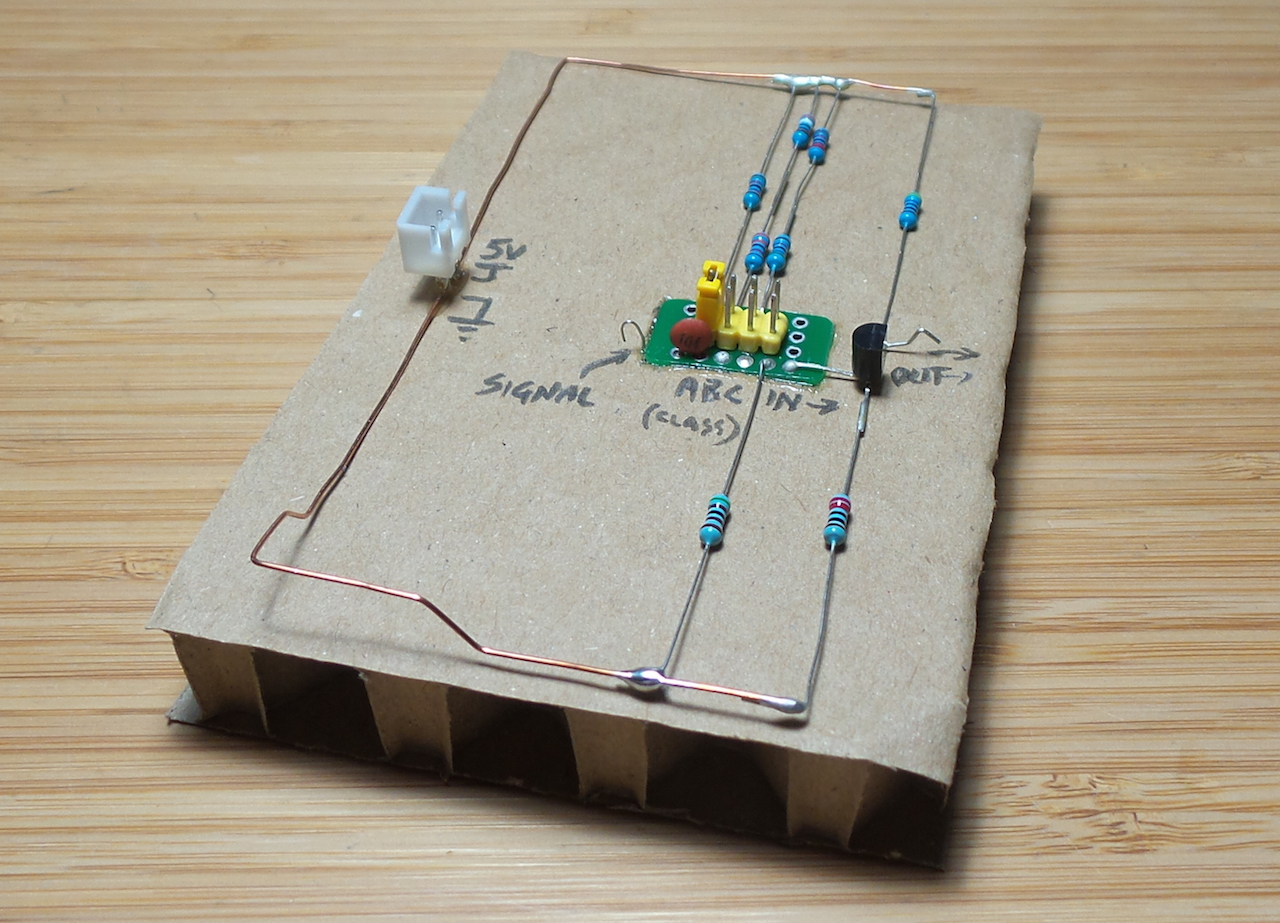Time to revisit the basics of biasing a bipolar junction transistor in an NPN common-emitter amplifier configuration. I am inspired once again by one of w2aew’s excellent vidoes - this time #113: Basics of Transistor bias point and the class of amplifier operation.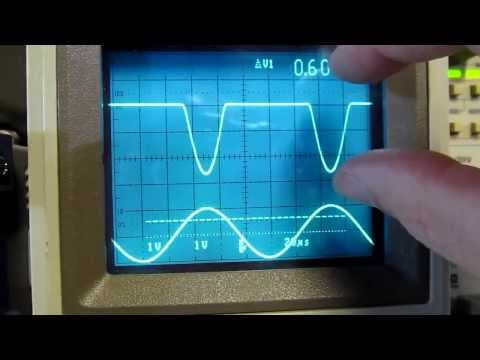### The Common Emitter Amplifier

The common emitter (CE) amplifier arrangement refers to cases where the transistor emitter shares a connection to both the input and output signal (ignoring resistors that may be in the path).

CE amplifiers generally have:

• “modest” gain
• input impedence of a few kΩ
• inverted output

Biasing the amplifier aims to place the transistor somewhere in the active region, between cut-off and saturation. Specifically, this means setting the:

• DC operating point (Quiescent Point) with no applied input signal
• gain

Together these will determine the class of operation.

### Class of Operation

Class Amplifies Typical Applications
A entire waveform without distortion (360˚) high fidelity linear audio amplifiers
B half cycle (180˚) RF
C less than half cycle (< 180˚) oscillator circuits
AB between half and full cycle (180˚-360˚) audio power amplifiers

It may seem like class A should always be preferred, but that is not true as it is also the most power hungry.

### Design Steps

An approach and example for selecting values for a simple CE amplifier:

#### 1. Choose the operating requirements:

• VCC = 5V
• A = 2 (low gain)
• quiescent current Icq = 4mA (a value to keep power dissipation low)
• quescent voltage Vceq = 2.5 V (rule of thumb - about half VCC)
• assume ß (hFE) = 150 (or lookup the datasheet)
• assume Vbe = 0.7V (or lookup the datasheet)

#### 2. calculate collector + emitter resistance for desired gain at the Q point

Aiming for Vcc/2

• Rc + Re = (5V/2) / 4mA = 625Ω

#### 3. calculate Rc and Re for desired gain

• A ≅ Rc/Re
• Re = 625Ω - Rc
• Rc = 2 * 625Ω - 2 * Rc
• Rc = 2/3 * 625Ω
• Re = 1/3 x 625Ω = 208Ω, say 220Ω (standard value)
• Rc = 416Ω, say 470Ω (standard value)

#### 5. calculate the combined bias gang resistance

assume current through the gang at 10 x Ib as a rule of thumb to ensure “stiff” biasing i.e. 0.2667mA

so combined resistance = `5V/0.2667mA` = 18.8kΩ

#### 6. calculate the resistance of R1 and R2 components of the bias gang

Lower resistor R2:

voltage = 0.7 + Ic x Re = 1.58V

therefore R2 = 5924Ω so choose 5 kΩ (standard value)

and therefore R1 = 13.8kΩ so choose 12kΩ (standard value)

#### 7. review input limits

with a design gain of 2, and assuming we have say 4V peak-to-peak headroom around the 2.5V quiesent point, we should be able to handle signals of 2V peak-to-peak

That’s all pretty theoretical and assumes nothing much about the transistor performance (except for ß), so let’s see how it works in practice.

With a 10kz 0.8V peak-to-peak input, here’s how I see the output on a scope.

• CH1: input (AC coupled)
• CH2: output (AC coupled)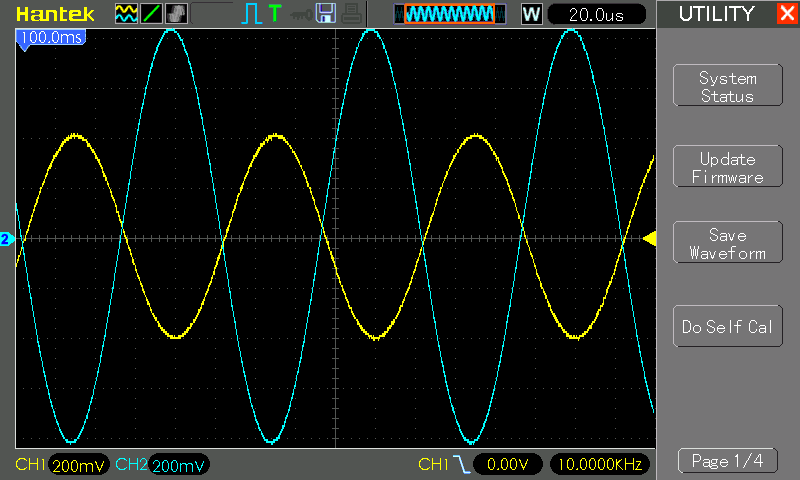That’s pretty spot-on!

• input bias point is around 1.48V, actually measures 816mV peak-to-peak on the scope
• output is centered on 3.12 V, and measures 1.68V peak-to-peak
• so an actual gain of 2.06
• no distortion - nice clean class A amplification

### Bias Class Testing

Borrowing heavily from w2aew’s tutorial, I’ve wired up a circuit to demonstrate the different classes of operation by switching R1.

#### Class B Operation

For class B (half waveform), we want the bias point to sit at around 0.6 to 0.7 V (the Vbe voltage drop).

Keeping R2 at 5kΩ, we should switch R1 to around 37kΩ to scale the bias point.

Here’s the result. Just about perfect.

• CH1: input (DC coupled)
• CH2: output (DC coupled and offset -5V)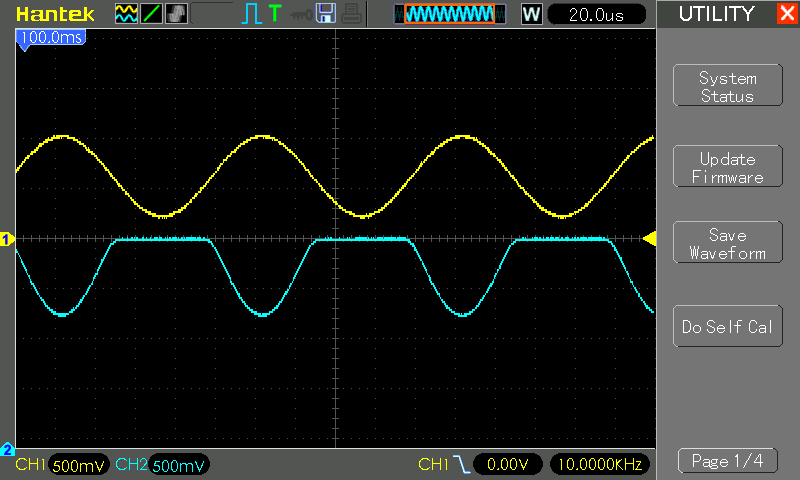Note: I didn’t scale R1 and R2 back accordingly to keep the current through the bias gang above 10 x Ib.

#### Class C Operation

For class C (less that half waveform), I just increased and adjusted R1 by trial and error to get a minimal peak. Finally settled at R1 ~80kΩ.

• CH1: input (DC coupled)
• CH2: output (DC coupled and offset -5V)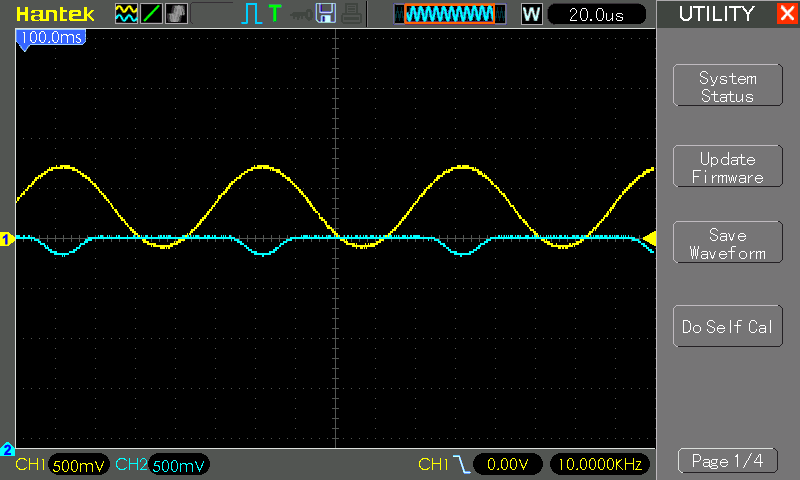Note: I didn’t scale R1 and R2 back accordingly to keep the current through the bias gang above 10 x Ib.

### Input and Output Impedence Calculation

Input impedance:

• the input sees R1, R2 and the impedence of the base (about 33k, hFE * Re) in parallel, so around 5kΩ
• the input capacitor combines with the resistance in a high-pass filter, C1 should be chosen to ensure input frequencies are far above the 3dB point

Output impedence:

• just Rc in parallel with the impedence looking into the collector, which is “very large”
• so Rc is a good approximation i.e. 470Ω in this case

## Bypassed Emitter Resistor and Other Refinements

It is common to see a bypass capacitor in parallel with the emittor resistor. This improves stability of a grounded emitter amplifier i.e. when Re is low to maximise gain. No calculations or experiments for that here yet.

In practice, biasing can get a whole lot more complex, and “real” amplifier circuits may involve multiple transistors, either in Darlington or push-pull configurations, with biasing tricks that involve diodes to fix particular voltage drops.

I first breadboarded this experiment, and used an external function generator for the 10kHz input signal.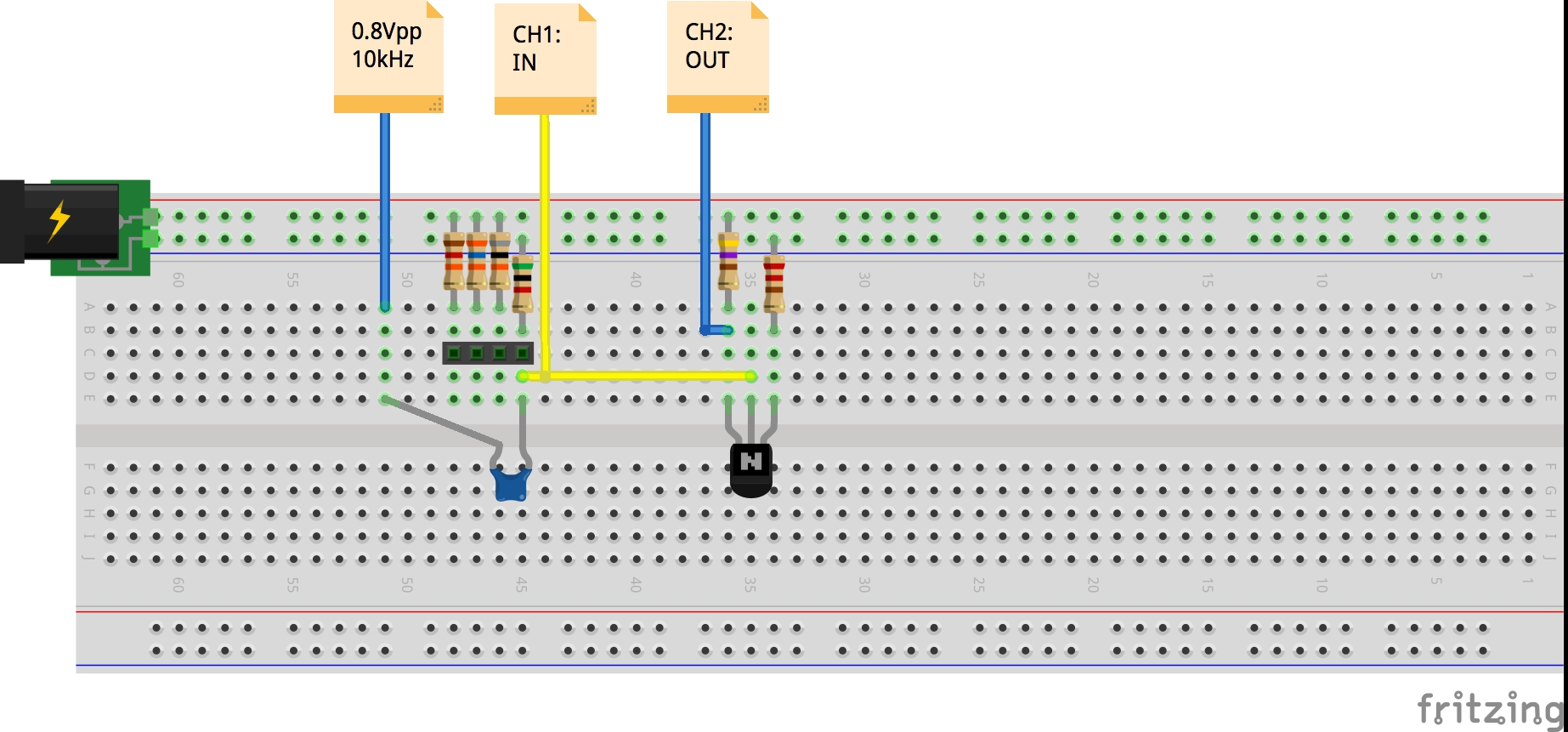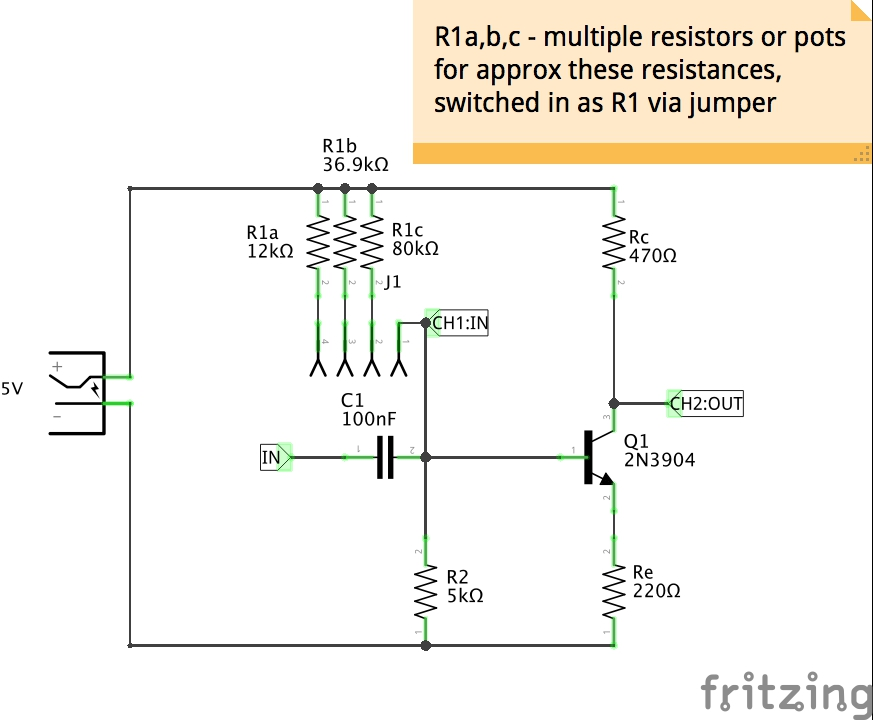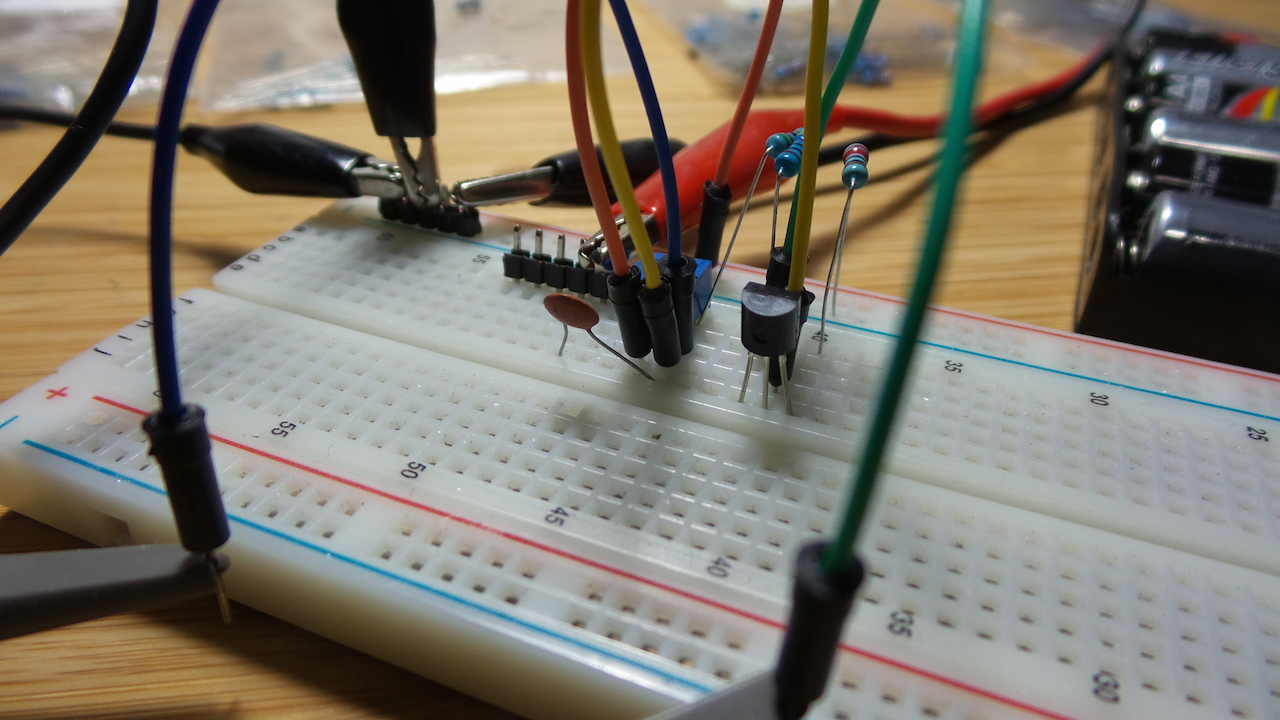## Ugly Demo Board

Just for fun, I mounted the circuit ugly style on some discarded packaging. A jumper is used to select from the pre-set Class A, B, C configurations.Under test, performs just fine..